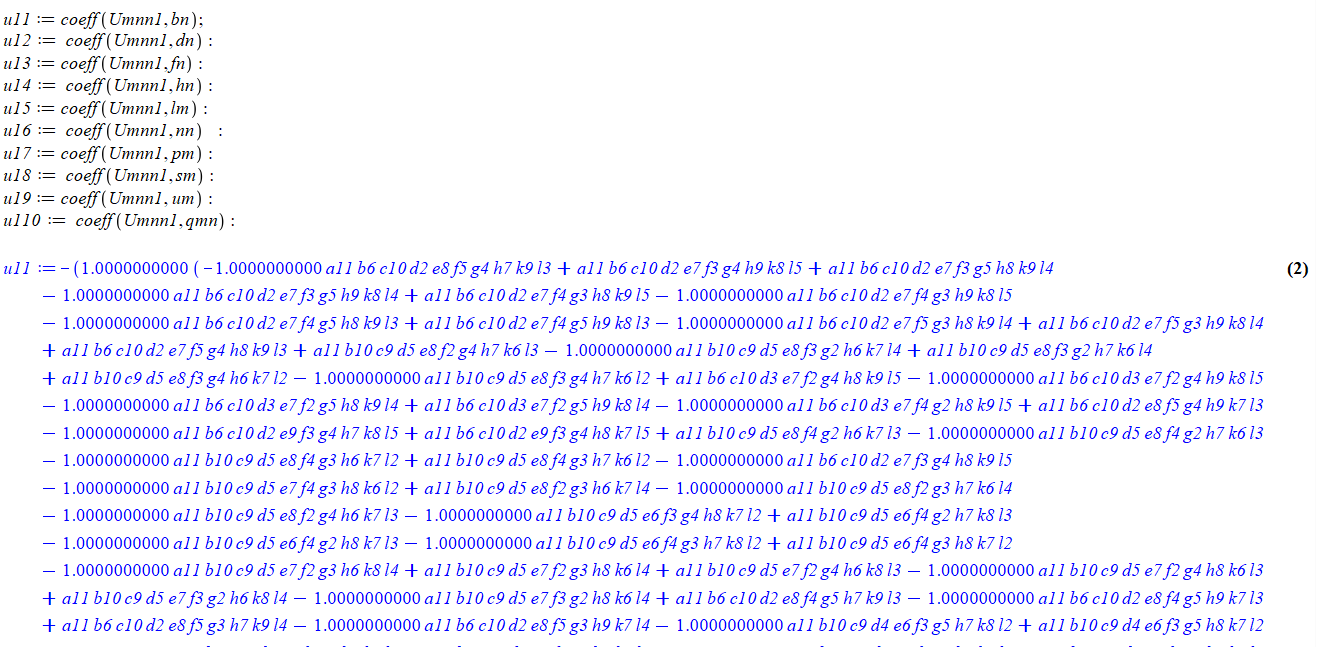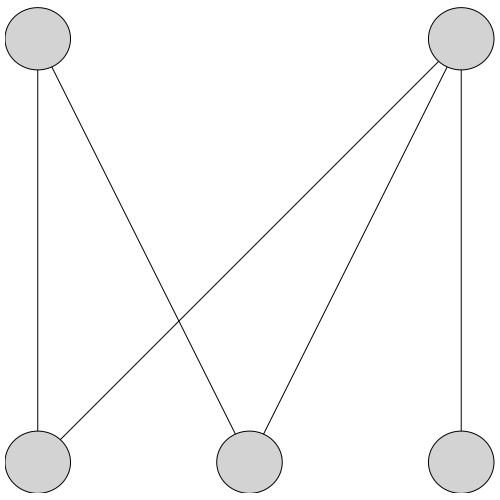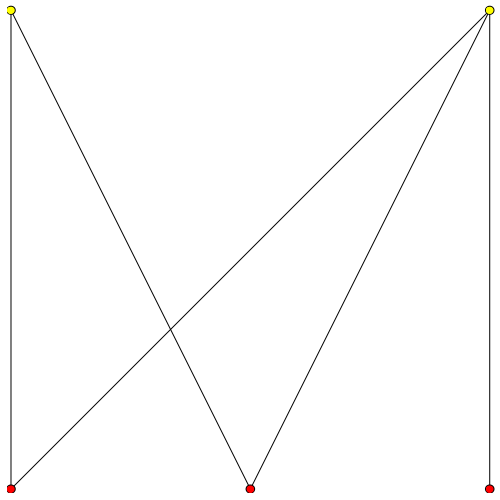## Maple is giving "Error, (in fprintf) string expect...

Hello,

I am collecting my coefficients with the below code. Since it is a huge data, i just give a some partion. So I want to write u11 to a text file. But when i use this command  save u11, "u111.txt" . It is writing to text but with big blanks. I show you at the below picture. So when i put it to matlab, it is again with spaces and matlab giving errors. So how can i save my long symbolic output (you can see in picture)  to a text file without spaces. I used

fd := "C:/double/u11.txt"

fprintf(fd, "%s", u11)

fclose(fd)

But it did not work. So if you could help me, i really appreciate. My best regards.## Will anyone be able to speed up this code even mor...

A robust largescale index-1 DAE (and stiff ODE) solver has been developed in Maple.

PS: These codes run only with the Java version of Maple. Codes are saved as *.mws for the ease of typing. One can download the files and save them as *.mw as well.
Details about our approach are provided in a paper just submitted.
See arxiv at
https://arxiv.org/abs/2212.02630

We encourage everyone to test these codes and report bugs. All the examples can be run with Intel's Pardiso (provided users have access to libraries) by calling DAESolverP.txt and by calling "IMPDAEP" instead of "IMPDAE". This is useful for large-scale problems. The symbolic capability and ListTools search capability of Maple are very good and can be used for developing optimization solvers as well.

I would like to know if CPU time/memory usage can be reduced significantly. In particular,  for examples 5 and 6. Some ways to contribute include

(1) Running the code in evalhf or compiled form. This may be hard.
(2) Providing options to run other parallel open-source linear solvers (eg., MUMPS).

(3) Other examples that show the use of the developed solver. We are able to solve > 100,000 DAEs.

(4) Helping in converting the code to Maple 14 or earlier (by doing sparse LU Decomposition. Just using LinearSolve will slow down the code).

Please avoid ~,*, etc (shortcuts) unless it improves the speed of calculation.
Thanks
Dr. Venkat Subramanian

mw format files are given below

## Drawing large vertices with chromatic number...

With
DrawGraph(G, stylesheet = [vertexpadding = 35], showlabels = false)
I get thisBut if I wish emphasize the chromatic number, with
COLORS := [red, yellow, cyan, green, coral, blue, magenta, navy, orange, pink, plum, khaki, turquoise];
cn := ChromaticNumber(G, 'col');
for i to cn do
HighlightVertex(G, col[i], COLORS[i]);
end do;
DrawGraph(G, stylesheet = [vertexpadding = 35], showlabels = false);
I get thisI want bigger vertices, any suggestions? Thanks

## I need this plot...

> expr[[(-.684-1.08*r+6.1*r^2-2*r^3+0.36e-1*ln(10.*r)-.3*r^2*ln(10.*r))/(-1.14+1.2*r+0.6e-1*ln(10.*r)), 1.290994449*sqrt((-.1296*r+1.512*r^2-.36*r^3+6.1*r^4-2*r^5-0.72e-1*r^2*ln(10.*r)-.3*r^4*ln(10.*r)+(-.684-1.08*r+6.1*r^2-2*r^3+0.36e-1*ln(10.*r)-.3*r^2*ln(10.*r))*(.216*r-2.52*r^2+.12*r^2*ln(10.*r))/(-1.14+1.2*r+0.6e-1*ln(10.*r)))/(-1.14+1.2*r+0.6e-1*ln(10.*r)))], [(-.684-1.08*r+6.1*r^2-2*r^3+0.36e-1*ln(10.*r)-.3*r^2*ln(10.*r))/(-1.14+1.2*r+0.6e-1*ln(10.*r)), -1.290994449*sqrt((-.1296*r+1.512*r^2-.36*r^3+6.1*r^4-2*r^5-0.72e-1*r^2*ln(10.*r)-.3*r^4*ln(10.*r)+(-.684-1.08*r+6.1*r^2-2*r^3+0.36e-1*ln(10.*r)-.3*r^2*ln(10.*r))*(.216*r-2.52*r^2+.12*r^2*ln(10.*r))/(-1.14+1.2*r+0.6e-1*ln(10.*r)))/(-1.14+1.2*r+0.6e-1*ln(10.*r)))]];
> plot([seq([expr[j][], r = 0 .. 1], j = 1 .. 2)], -6 .. 6, -10 .. 10, color = [blue, blue]);

## How to simplify D-operator into f' form when do th...

Hi all,

When I am differentiate the composite functions, I wanna make the output more elegant.

For example, from the following code:

```restart;
A__1 := A__0 + k(e)*a__1;
diff(phi(A__1), a__1);
```

I define a function phi(A__1) and function A__1(a__1), and the latter is known.

But Maple output:

`D(numtheory:-phi)(A__0 + k(e)*a__1)*k(e)`

I think "D(numtheory:-phi)(A__0 + k(e)*a__1)" part can be simplified into d(phi)/d(A__1).

Do you have some ideas? Thanks!

## How do I get the result from a QuestionDialog - re...

Trying to use the following question dialog

with(Maplets[Elements]);
maplet := Maplet(QuestionDialog("Is x > 0?"));
result := parse(Maplets[Display](maplet));

Displays question with Yes and No buttons but whichever button is clicked the result is always false.  This was based closely on an example from the documentation.

So, how can I tell which button was clicked.  Also, the close button could have been clicked - how should that be dealt with?

## How/Can you insert a line between 2 others in work...

```In 1D worksheet mode I can't figure out how to insert a line between a and c.

restart

a:=1
a := 1

c:=3
c := 3

```

## How to Convert a Worksheet to a Document?...

Hello guys.

Is there any way to convert a worksheet to a document and vice versa?

Regards,

Oliveira.

## guideline regarding axis , its style and font etc...

Hello, everyone. I have plotted a simple equation and now have the following observation regarding it.

1- How to change or control axis style mean style like bold, colour change etc?

2-  How to change or control the font, colour and style of numbers written automatically by maple while plotting on the axis (x-axis, y-axis)?

3- How to change the font, style and colours of labels?

The problem I am facing is that whenever I save or import any graph as png or jpeg and then paste it into MS word, it's quality or pixel changes and it looks very bad. is there any way to save and paste good-quality pictures into documents that means DPI?

Qaulity_Help.mw

## Why does restart at beginning of Document break an...

I am trying to use procedures for the first time in a Document.  I copied and pasted an example (addList) from the documentation into my document.  This example works fine but putting a restart at the beginning of the Document breaks it and I don't understand why.

I am also trying to write my own very first procedure, more or less following the pattern of the example, but that is giving an unable to parse error and, again, I don't understand why.

I am sure this is a very simple error on my part but I would appreciate some help un understanding what I am doing wrong.

Thank you.

ProcsTests.mw

## Is Maple on Linux as reliable as on Mac?...

I've been running some animations on a Macbook Pro 2015 8GB RAM and a Macbook Pro SIlicon 2021 16GB RAM. The problem I was trying to solve was making these laptops run out of memory (animation of a 3D trajectory with 100000 points).

So I switched to a desktop linux setup with 256GB of RAM and 16 cores. I installed Maple and set everything up but it seems SUPER buggy (menus disappearing when I try to hover over their items) and it just crashes on versions of the problem I have that are easy to run even on the 8GB RAM Macbook from 2015.

Is there a history of the Linux version being worse than the Mac version or something? Is there any chance an .mla file I created on a Mac somehow doesn't work correct on Linux? Could it be some internal Maple limitation on memory? I am wondering what it could be.

The OS is Ubuntu 22.10 and the architecture is x86-64.

The requirements say Ubuntu 21.10. Does this mean at least 21.10, or exactly 21.10?

Maple is 2022.2.

Maple Build Number is 1657361

The freezing/crashing of Maple seems to occur when I play an animation. Everything gets slow and then everything stops working. 1000 point trajectory on a sphere. No issue on a much less powerful Macbook.